## ThinkDSP¶

This notebook contains code examples from Chapter 7: Discrete Fourier Transform

In :
# Get thinkdsp.py

import os

if not os.path.exists('thinkdsp.py'):
!wget https://github.com/AllenDowney/ThinkDSP/raw/master/code/thinkdsp.py

In :
import numpy as np
import matplotlib.pyplot as plt

from thinkdsp import decorate
PI2 = 2 * np.pi

In :
# suppress scientific notation for small numbers
np.set_printoptions(precision=3, suppress=True)


## Complex sinusoid¶

Here's the definition of ComplexSinusoid, with print statements to display intermediate results.

In :
from thinkdsp import Sinusoid

class ComplexSinusoid(Sinusoid):
"""Represents a complex exponential signal."""

def evaluate(self, ts):
"""Evaluates the signal at the given times.

ts: float array of times

returns: float wave array
"""
print(ts)
phases = PI2 * self.freq * ts + self.offset
print(phases)
ys = self.amp * np.exp(1j * phases)
return ys


Here's an example:

In :
signal = ComplexSinusoid(freq=1, amp=0.6, offset=1)
wave = signal.make_wave(duration=1, framerate=4)
print(wave.ys)

[0.   0.25 0.5  0.75]
[1.    2.571 4.142 5.712]
[ 0.324+0.505j -0.505+0.324j -0.324-0.505j  0.505-0.324j]


The simplest way to synthesize a mixture of signals is to evaluate the signals and add them up.

In :
from thinkdsp import SumSignal

def synthesize1(amps, freqs, ts):
components = [ComplexSinusoid(freq, amp)
for amp, freq in zip(amps, freqs)]
signal = SumSignal(*components)
ys = signal.evaluate(ts)
return ys


Here's an example that's a mixture of 4 components.

In :
amps = np.array([0.6, 0.25, 0.1, 0.05])
freqs = [100, 200, 300, 400]
framerate = 11025

ts = np.linspace(0, 1, framerate, endpoint=False)
ys = synthesize1(amps, freqs, ts)
print(ys)

[0. 0. 0. ... 1. 1. 1.]
[  0.      0.057   0.114 ... 628.148 628.205 628.262]
[0. 0. 0. ... 1. 1. 1.]
[   0.       0.114    0.228 ... 1256.295 1256.409 1256.523]
[0. 0. 0. ... 1. 1. 1.]
[   0.       0.171    0.342 ... 1884.443 1884.614 1884.785]
[0. 0. 0. ... 1. 1. 1.]
[   0.       0.228    0.456 ... 2512.59  2512.818 2513.046]
[1.   +0.j    0.995+0.091j 0.979+0.18j  ... 0.953-0.267j 0.979-0.18j
0.995-0.091j]


Now we can plot the real and imaginary parts:

In :
n = 500
plt.plot(ts[:n], ys[:n].real)
plt.plot(ts[:n], ys[:n].imag)
decorate(xlabel='Time')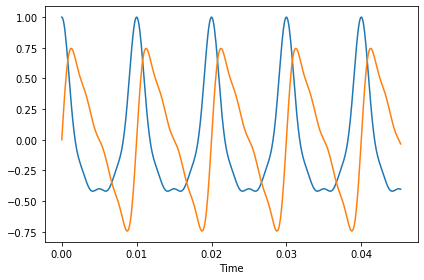The real part is a mixture of cosines; the imaginary part is a mixture of sines. They contain the same frequency components with the same amplitudes, so they sound the same to us:

In :
from thinkdsp import Wave

wave = Wave(ys.real, framerate)
wave.apodize()
wave.make_audio()

Out:
In :
wave = Wave(ys.imag, framerate)
wave.apodize()
wave.make_audio()

Out:

We can express the same process using matrix multiplication.

In :
def synthesize2(amps, freqs, ts):
args = np.outer(ts, freqs)
M = np.exp(1j * PI2 * args)
ys = np.dot(M, amps)
return ys


And it should sound the same.

In :
amps = np.array([0.6, 0.25, 0.1, 0.05])
ys = synthesize2(amps, freqs, ts)
print(ys)

[1.   +0.j    0.995+0.091j 0.979+0.18j  ... 0.953-0.267j 0.979-0.18j
0.995-0.091j]

In :
wave = Wave(ys.real, framerate)
wave.apodize()
wave.make_audio()

Out:

To see the effect of a complex amplitude, we can rotate the amplitudes by 1.5 radian:

In :
phi = 1.5
amps2 = amps * np.exp(1j * phi)
ys2 = synthesize2(amps2, freqs, ts)

n = 500
plt.plot(ts[:n], ys.real[:n], label=r'$\phi_0 = 0$')
plt.plot(ts[:n], ys2.real[:n], label=r'$\phi_0 = 1.5$')
decorate(xlabel='Time')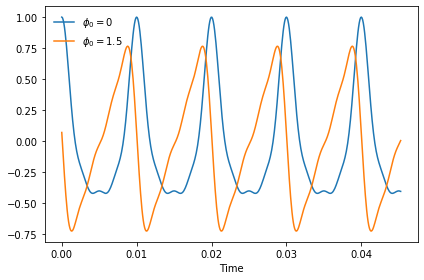Rotating all components by the same phase offset changes the shape of the waveform because the components have different periods, so the same offset has a different effect on each component.

### Analysis¶

The simplest way to analyze a signal---that is, find the amplitude for each component---is to create the same matrix we used for synthesis and then solve the system of linear equations.

In :
def analyze1(ys, freqs, ts):
args = np.outer(ts, freqs)
M = np.exp(1j * PI2 * args)
amps = np.linalg.solve(M, ys)
return amps


Using the first 4 values from the wave array, we can recover the amplitudes.

In :
n = len(freqs)
amps2 = analyze1(ys[:n], freqs, ts[:n])
print(amps2)

[0.6 +0.j 0.25-0.j 0.1 +0.j 0.05-0.j]


If we define the freqs from 0 to N-1 and ts from 0 to (N-1)/N, we get a unitary matrix.

In :
N = 4
ts = np.arange(N) / N
freqs = np.arange(N)
args = np.outer(ts, freqs)
M = np.exp(1j * PI2 * args)
print(M)

[[ 1.+0.j  1.+0.j  1.+0.j  1.+0.j]
[ 1.+0.j  0.+1.j -1.+0.j -0.-1.j]
[ 1.+0.j -1.+0.j  1.-0.j -1.+0.j]
[ 1.+0.j -0.-1.j -1.+0.j  0.+1.j]]


To check whether a matrix is unitary, we can compute $M^* M$, which should be the identity matrix:

In :
MstarM = M.conj().transpose().dot(M)
print(MstarM.real)

[[ 4. -0.  0.  0.]
[-0.  4. -0.  0.]
[ 0. -0.  4. -0.]
[ 0.  0. -0.  4.]]


The result is actually $4 I$, so in general we have an extra factor of $N$ to deal with, but that's a minor problem.

We can use this result to write a faster version of analyze1:

In :
def analyze2(ys, freqs, ts):
args = np.outer(ts, freqs)
M = np.exp(1j * PI2 * args)
amps = M.conj().transpose().dot(ys) / N
return amps

In :
N = 4
amps = np.array([0.6, 0.25, 0.1, 0.05])
freqs = np.arange(N)
ts = np.arange(N) / N
ys = synthesize2(amps, freqs, ts)

amps3 = analyze2(ys, freqs, ts)
print(amps3)

[0.6 +0.j 0.25-0.j 0.1 -0.j 0.05-0.j]


Now we can write our own version of DFT:

In :
def synthesis_matrix(N):
ts = np.arange(N) / N
freqs = np.arange(N)
args = np.outer(ts, freqs)
M = np.exp(1j * PI2 * args)
return M

In :
def dft(ys):
N = len(ys)
M = synthesis_matrix(N)
amps = M.conj().transpose().dot(ys)
return amps


And compare it to analyze2:

In :
print(dft(ys))

[2.4+0.j 1. -0.j 0.4-0.j 0.2-0.j]


The result is close to amps * 4.

We can also compare it to np.fft.fft. FFT stands for Fast Fourier Transform, which is an even faster implementation of DFT.

In :
print(np.fft.fft(ys))

[2.4+0.j 1. -0.j 0.4-0.j 0.2-0.j]


The inverse DFT is almost the same, except we don't have to transpose $M$ and we have to divide through by $N$.

In :
def idft(ys):
N = len(ys)
M = synthesis_matrix(N)
amps = M.dot(ys) / N
return amps


We can confirm that dft(idft(amps)) yields amps:

In :
ys = idft(amps)
print(dft(ys))

[0.6 +0.j 0.25-0.j 0.1 -0.j 0.05-0.j]


### Real signals¶

Let's see what happens when we apply DFT to a real-valued signal.

In :
from thinkdsp import SawtoothSignal

framerate = 10000
signal = SawtoothSignal(freq=500)
wave = signal.make_wave(duration=0.1, framerate=framerate)
wave.make_audio()

Out:

wave is a 500 Hz sawtooth signal sampled at 10 kHz.

In :
hs = dft(wave.ys)
len(wave.ys), len(hs)

Out:
(1000, 1000)

hs is the DFT of this wave, and amps contains the amplitudes.

In :
amps = np.abs(hs)
plt.plot(amps)
decorate(xlabel='Frequency (unspecified units)', ylabel='DFT')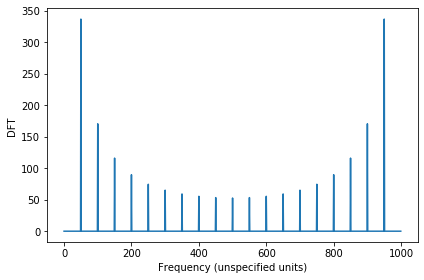The DFT assumes that the sampling rate is N per time unit, for an arbitrary time unit. We have to convert to actual units -- seconds -- like this:

In :
N = len(hs)
fs = np.arange(N) * framerate / N


Also, the DFT of a real signal is symmetric, so the right side is redundant. Normally, we only compute and plot the first half:

In :
plt.plot(fs[:N//2+1], amps[:N//2+1])
decorate(xlabel='Frequency (Hz)', ylabel='DFT')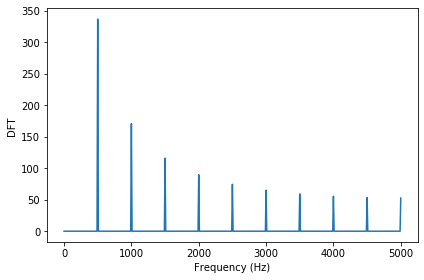Let's get a better sense for why the DFT of a real signal is symmetric. I'll start by making the inverse DFT matrix for $N=8$.

In :
M = synthesis_matrix(N=8)


And the DFT matrix:

In :
Mstar = M.conj().transpose()


And a triangle wave with 8 elements:

In :
from thinkdsp import TriangleSignal

wave = TriangleSignal(freq=1).make_wave(duration=1, framerate=8)
wave.ys

Out:
array([ 1. ,  0.5,  0. , -0.5, -1. , -0.5,  0. ,  0.5])

Here's what the wave looks like.

In :
wave.plot()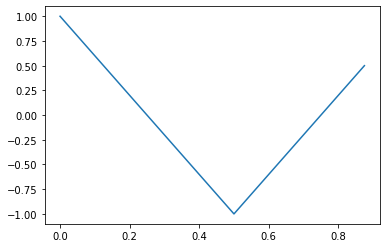Now let's look at rows 3 and 5 of the DFT matrix:

In :
row3 = Mstar[3, :]
print(row3)

[ 1.   -0.j    -0.707-0.707j -0.   +1.j     0.707-0.707j -1.   -0.j
0.707+0.707j  0.   -1.j    -0.707+0.707j]

In :
row5 = Mstar[5, :]
row5

Out:
array([ 1.   -0.j   , -0.707+0.707j,  0.   -1.j   ,  0.707+0.707j,
-1.   -0.j   ,  0.707-0.707j, -0.   +1.j   , -0.707-0.707j])

They are almost the same, but row5 is the complex conjugate of row3.

In :
def approx_equal(a, b, tol=1e-10):
return np.sum(np.abs(a-b)) < tol

In :
approx_equal(row3, row5.conj())

Out:
True

When we multiply the DFT matrix and the wave array, the element with index 3 is:

In :
X3 = row3.dot(wave.ys)
X3

Out:
(0.5857864376269053-1.322063213371145e-16j)

And the element with index 5 is:

In :
X5 = row5.dot(wave.ys)
X5

Out:
(0.585786437626906-5.534107762827377e-16j)

And they are the same, within floating point error.

In :
abs(X3 - X5)

Out:
7.881290833695066e-16

Let's try the same thing with a complex signal:

In :
wave2 = ComplexSinusoid(freq=1).make_wave(duration=1, framerate=8)
plt.plot(wave2.ts, wave2.ys.real)
plt.plot(wave2.ts, wave2.ys.imag)

[0.    0.125 0.25  0.375 0.5   0.625 0.75  0.875]
[0.    0.785 1.571 2.356 3.142 3.927 4.712 5.498]

Out:
[<matplotlib.lines.Line2D at 0x7f0d02089f98>]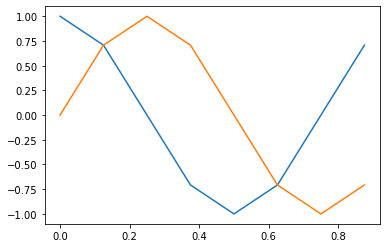Now the elements with indices 3 and 5 are different:

In :
X3 = row3.dot(wave2.ys)
X3

Out:
(1.7763568394002505e-15-2.6522243254460964e-16j)
In :
X5 = row5.dot(wave2.ys)
X5

Out:
(-2.220446049250313e-16+4.0860826919976536e-16j)

Visually we can confirm that the FFT of the real signal is symmetric:

In :
hs = np.fft.fft(wave.ys)
plt.plot(abs(hs))

Out:
[<matplotlib.lines.Line2D at 0x7f0d01fa3550>]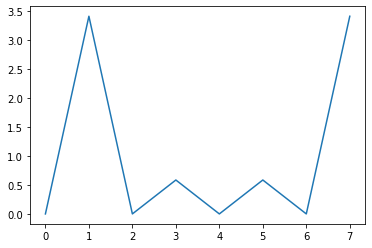And the FFT of the complex signal is not.

In :
hs = np.fft.fft(wave2.ys)
plt.plot(abs(hs))

Out:
[<matplotlib.lines.Line2D at 0x7f0d01f89da0>]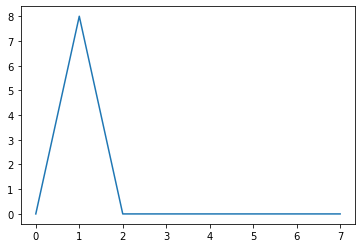Another way to think about all of this is to evaluate the DFT matrix for different frequencies. Instead of $0$ through $N-1$, let's try $0, 1, 2, 3, 4, -3, -2, -1$.

In :
N = 8
ts = np.arange(N) / N
freqs = np.arange(N)
freqs = [0, 1, 2, 3, 4, -3, -2, -1]
args = np.outer(ts, freqs)
M2 = np.exp(1j * PI2 * args)

In :
approx_equal(M, M2)

Out:
True

So you can think of the second half of the DFT as positive frequencies that get aliased (which is how I explained them), or as negative frequencies (which is the more conventional way to explain them). But the DFT doesn't care either way.

The thinkdsp library provides support for computing the "full" FFT instead of the real FFT.

In :
framerate = 10000
signal = SawtoothSignal(freq=500)
wave = signal.make_wave(duration=0.1, framerate=framerate)

In :
spectrum = wave.make_spectrum(full=True)

In :
spectrum.plot()
decorate(xlabel='Frequency (Hz)', ylabel='DFT')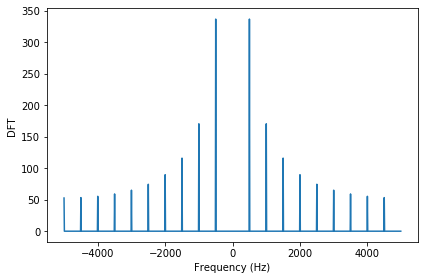In [ ]: